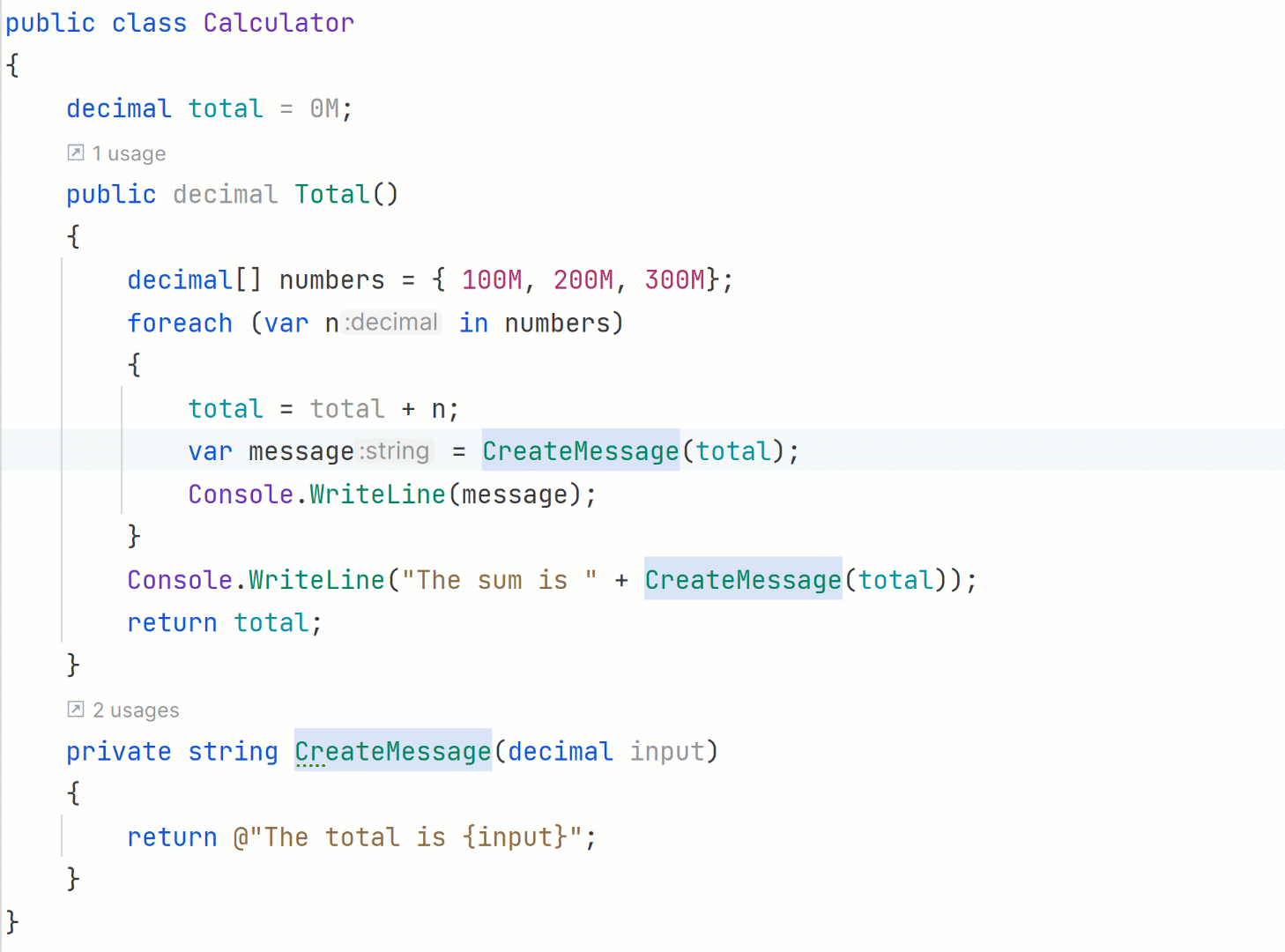## Inline method refactoring

### Replace usages of a function with its implementation.Inline Method refactoring helps you replace usages of a static, instance, and extension method with its body, and optionally remove the original method declaration.

This means that sometimes you need to un-DRY your code just a little. The point of DRY code is so we not only avoid exact duplication of code but duplication of its behaviors or output as well, even if the code isn't exactly the same. In practice, this might mean that you've refactored a method all the way down to one line. But there are still many calls to that one line function in the code. This is a good time to roll that function's one-line body into the calls with Inline Method reactorings.

Let's look at a `Calculate` method, before and after refactoring. Since the `CreateMessage` only prints out the total, its single-line body can easily replace the calls to it. In this case there is no need for an extra function for a one line call.

Before:

``public class Calculator{  decimal total = 0M;  public decimal Total()  {    decimal[] numbers = { 100M, 200M, 300M};    foreach (var n in numbers)    {      total = total + n;      var message = CreateMessage(total);      Console.WriteLine(message);    }    Console.WriteLine("The sum is " + CreateMessage(total));    return total;  }  private string CreateMessage(decimal input)  {    return @"The total is {input}";  }}``

After:

``public class Calculator{  decimal total = 0M;  public decimal Total()  {    decimal[] numbers = { 100M, 200M, 300M};    foreach (var n in numbers)    {      total = total + n;      var message = @"The total is {input}";      Console.WriteLine(message);    }    Console.WriteLine("The sum is " + @"The total is {input}");    return total;  }}``

As the sample shows, there is less code after refactoring. It's clearer to read and therefore easier to maintain. Sometimes, less is more, and inline refactorings can help with this.